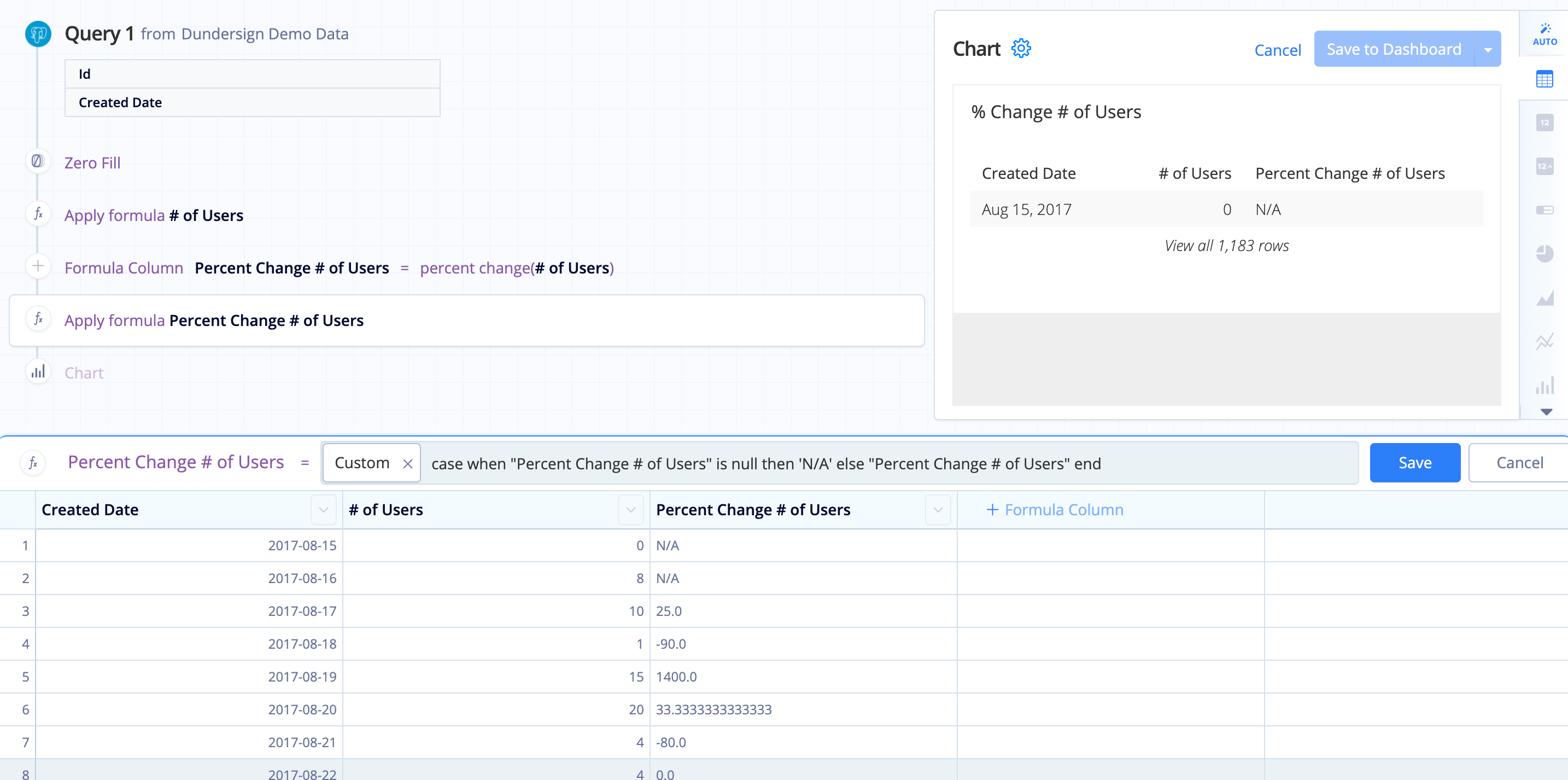# Calculate a percentage in Visual SQL

Percentages can be used to gain valuable insight into the affects a portion has on a total. They’re heavily relied upon to determine and evaluate KPIs for an organization and guide operations to improve your overall business performance.

In this example, using the SaaS Company Demo Data data source, we divide our cancelled subscriptions over our total subscriptions to calculate our churn rate. Churn rate is a common metric used by SaaS companies to determine how effectively they’re retaining their customers.

1. First, create a query to calculate the number of customers who have not cancelled. To do this, count the number of subscriptions where the Payment Start Date is not null and the Cancelled Date is null.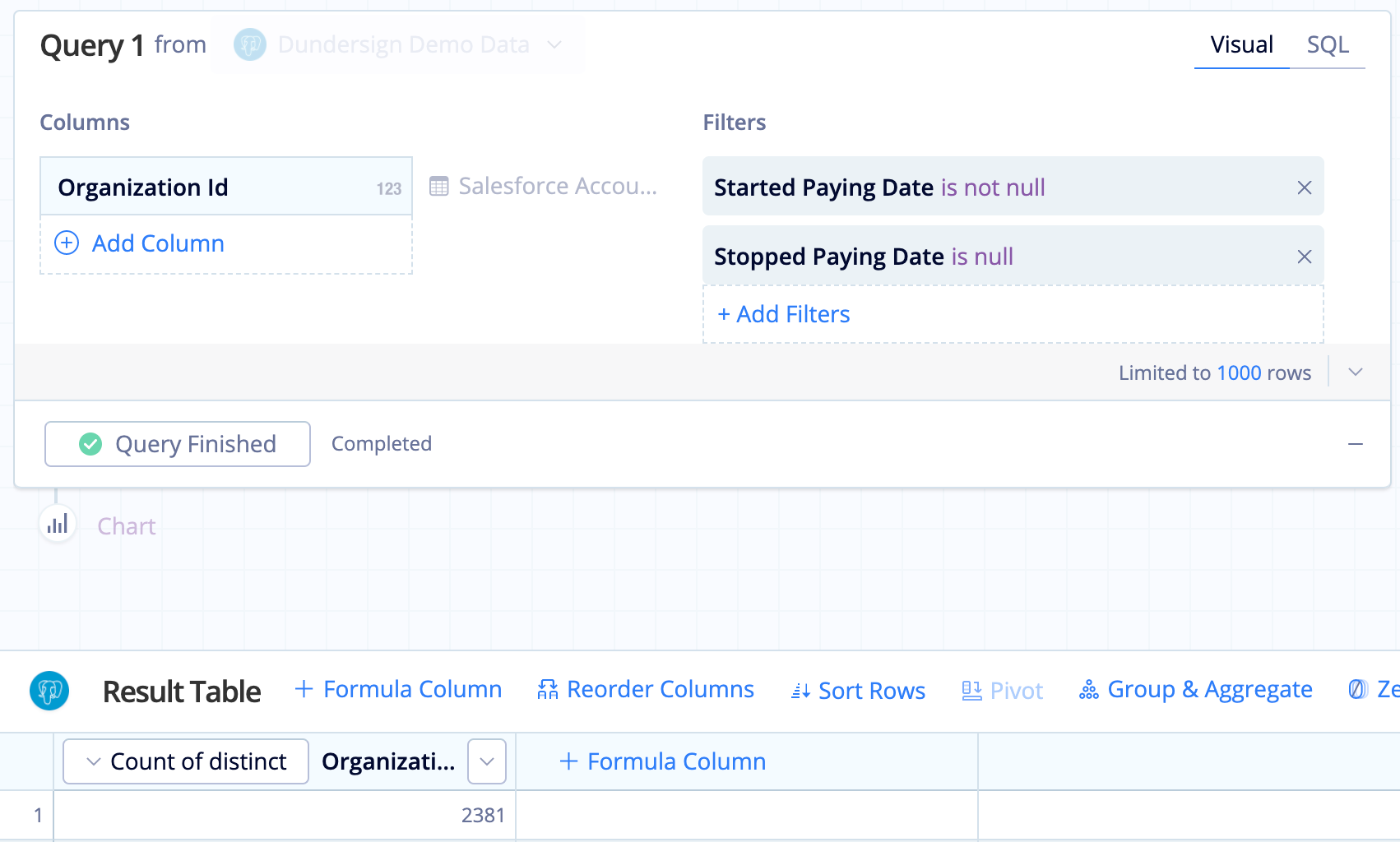2. Rename the column to `Current Subscriptions` for clarity.

3. Now add another query to get the count of subscriptions that have cancelled. To achieve this, count the number of subscriptions where both the Payment Start Date and the Cancelled Date are null.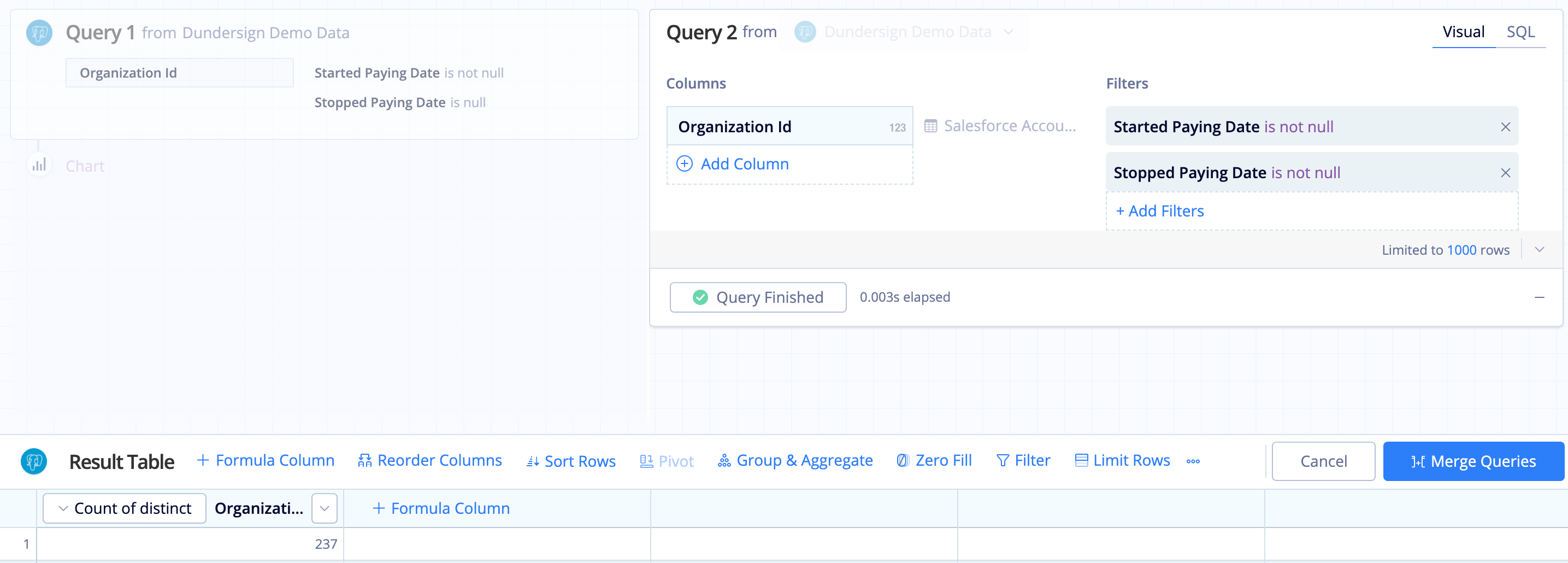4. Rename the column to `Cancelled Subscriptions` for clarity.

5. After creating the two queries, make sure to use a Cross Join when merging them.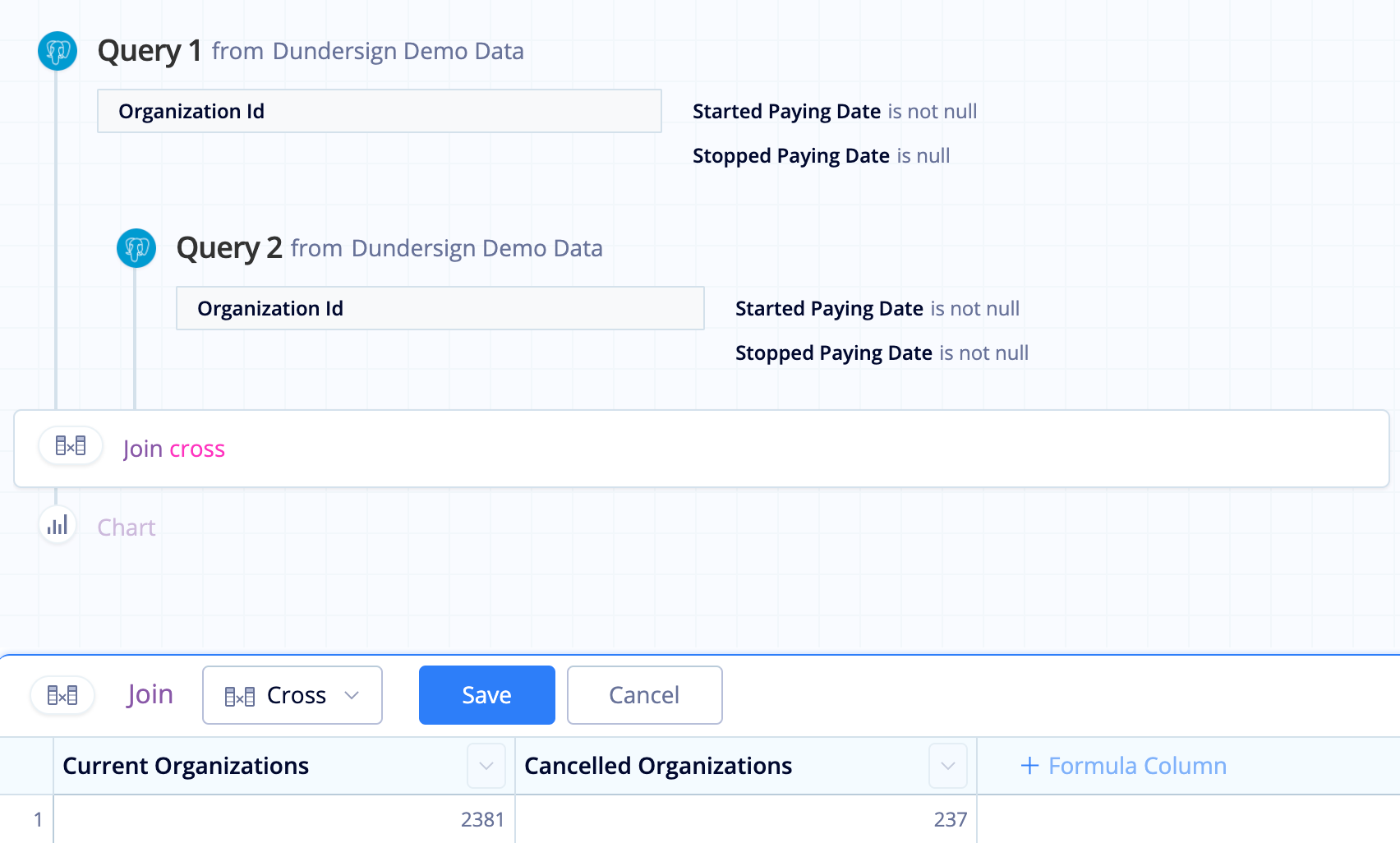6. Add a Formula Column, choose Custom for the formula type, then enter the following percentage formula:

`````` 100.0 * "Cancelled Subscriptions" / "Current Subscriptions"
``````

If you want to round your percentage to two decimal points, you can also use the SQLite `round()` function; the formula will look like this:

`````` round(100.0 * "Cancelled Subscriptions" / "Current Subscriptions", 2)
``````

where `2` is the number of decimal places.

Click Save to calculate the percentage.

Note: If your column values are integers and you’d like your results to have decimal precision, be sure to multiply the numerator by `100.0` to convert the column to a float data type. This converts the top value to a decimal before the division step and ensures your results are in decimal form.

7. Rename the Custom formula column to `Churn` for clarity.

The final result set should look like this: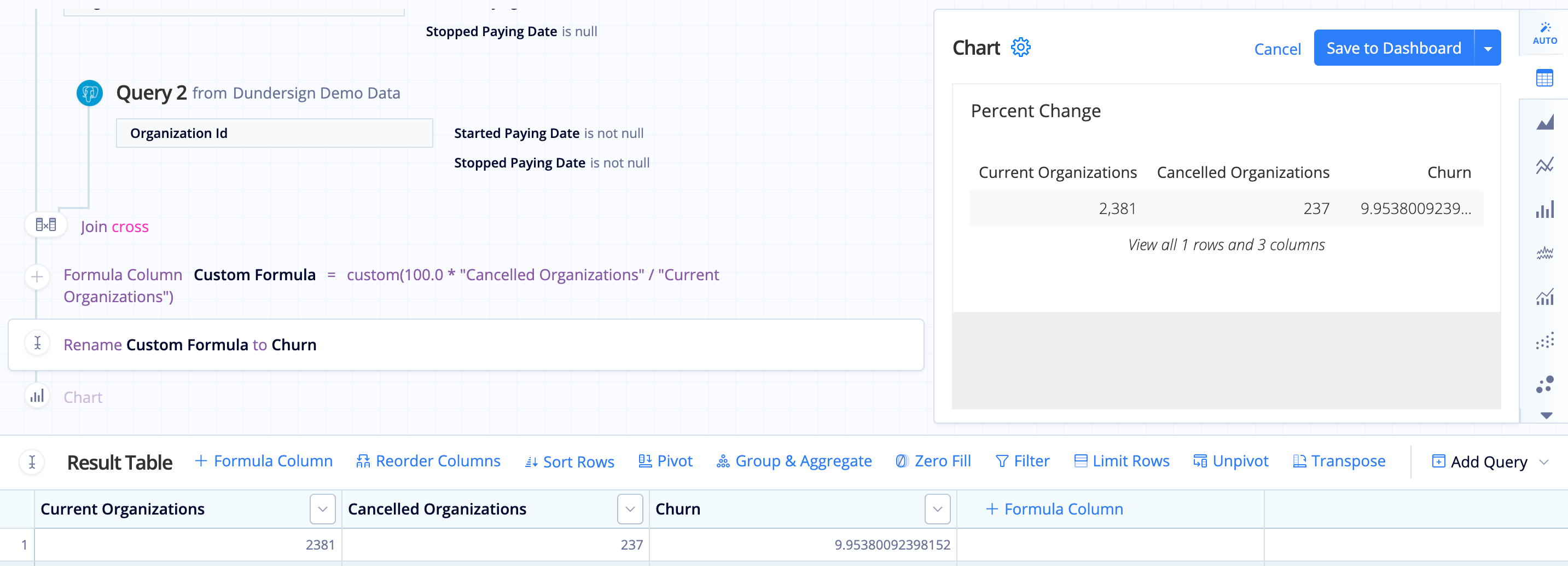## Troubleshoot Percent Change

If you’re using the Percent Change formula in your Pipeline, you may notice that if the previous row is 0, the percent change returns a NULL result. Don’t worry—this is expected, and we’ll help you deal with those situations.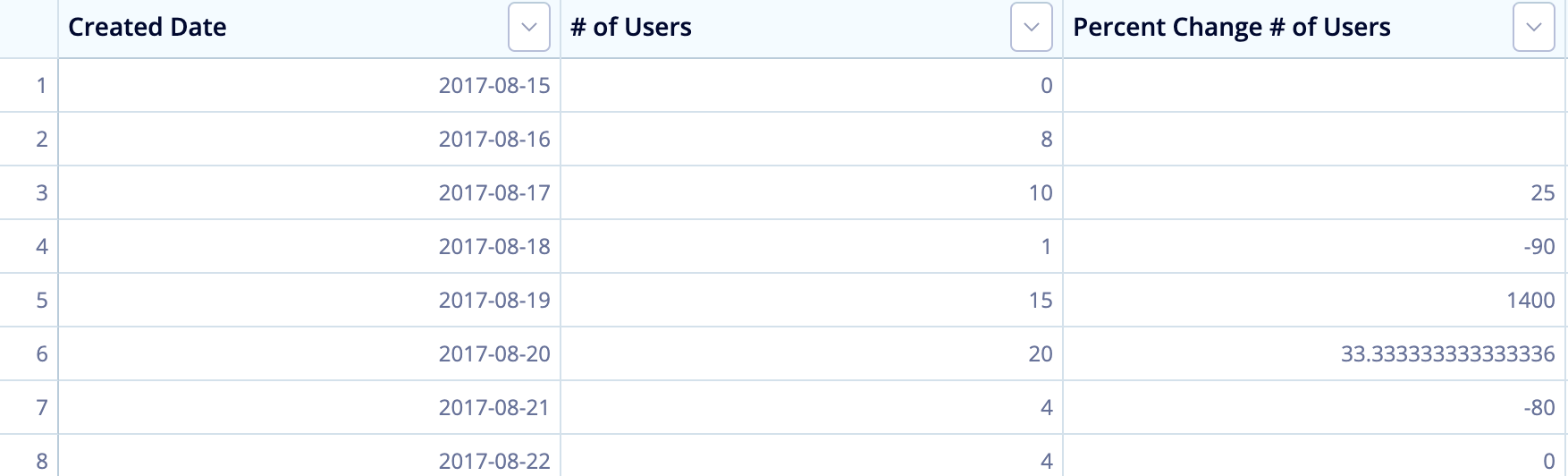Our Pipeline runs on SQLite, which adheres to Postel’s Rule. The default behavior when dividing by zero is to return NULL rather than throw an error when dividing by zero (see SQLite documentation for more information). This lines up with the generally accepted principle that the concept of percentage change has essentially no meaning when starting from zero.

There are several articles online showing potential workarounds. In Chartio, however, one workaround is to add an Edit Formula Action after the Percent Change to denote the percent change doesn’t apply here. You’d select a Custom formula and add the following CASE statement to it, replacing `Percent Change` with the name of your column:

``````case when "Percent Change" is null then 'N/A' else "Percent Change" end
``````